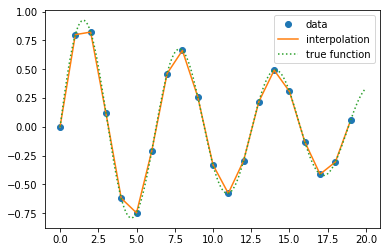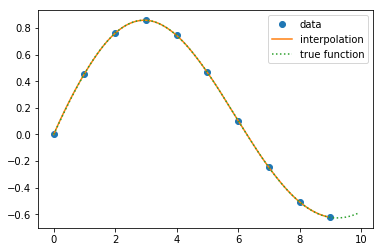Interpolation with scipy¶

Given a set of ordered discrete datapoints (the ordering is on the x axis, y values can be arbitrary), interpolation connects two neighboring nodes in such a way that the interpolation function goes right through those two datapoints.
This is different from linear or polynomial fit, where the fitting function just approximates bunch of discrete datapoints with one line (or curve). Hence it is not necessary that every datapoint would lie on the fitting function (it is rather rare actually for real life data).

Most common interpolation techniques are:

• linear interpolation
• polynomial interpolation
• spline interpolation

Scipy.interpolate library provides interp1d function, we call it like:

• f1 = interp1d(x, y, kind = 'linear')
• f2 = interp1d(x, y, kind = 'quadratic')
• f2 = interp1d(x, y, kind = 'cubic')

Linear interpolation:¶

In :
import matplotlib.pyplot as plt
from scipy import interpolate
import numpy as np
In :
x = np.arange(0, 20)
y = np.sin(x) * np.exp(-x/20.0)

# interpolation function from scipy
f = interpolate.interp1d(x, y, kind = 'linear')

# x range for the interpolation function
x_f = np.arange(0, 19, 0.1)
y_f = f(x_f)   # use interpolation function returned by interp1d

# true function that is being interpolated
x_t = np.arange(0, 20, 0.1)
y_t = np.sin(x_t) * np.exp(-x_t/20.0)

plt.plot(x, y, 'o', x_f, y_f, '-', x_t, y_t, ':')
plt.legend(['data', 'interpolation', 'true function'])

plt.show()Linear interpolation is rather crude and choppy, especialy for data with relatively distant points.

In [ ]:
import matplotlib.pyplot as plt
from scipy import interpolate
import numpy as np
In :
x = np.arange(0, 10)
y = np.sin(x/2.0) * np.exp(-x/20.0)

# interpolation function from scipy
f = interpolate.interp1d(x, y, kind = 'quadratic')

# x range for the interpolation function
x_f = np.arange(0, 9, 0.1)
y_f = f(x_f)   # use interpolation function returned by interp1d

# true function that is being interpolated
x_t = np.arange(0, 10, 0.1)
y_t = np.sin(x_t/2.0) * np.exp(-x_t/20.0)

plt.plot(x, y, 'o', x_f, y_f, '-', x_t, y_t, ':')
plt.legend(['data', 'interpolation', 'true function'])

plt.show()In the example above we can see that quadratic spline fits data very nicely.